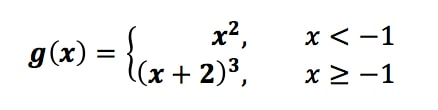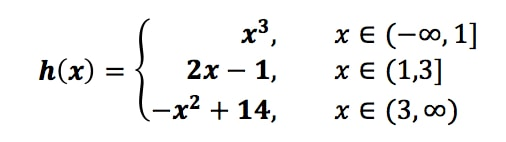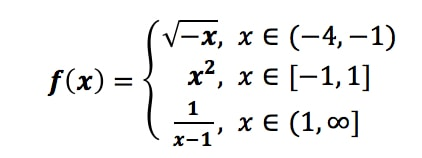# Graphing piecewise non-linear functions

### Graphing piecewise non-linear functions

#### Lessons

• 1.
For the following functiona)
Sketch the graph

b)
State the domain

c)
State the range

• 2.
For the following functiona)
Sketch the graph

b)
State the domain

c)
State the range

• 3.
For the following functiona)
Sketch the graph

b)
State the domain

c)
State the range

• 4.
a)
Graph $f(x) = |(x + 2)^{2} - 9|$; and

b)
Express $f(x) = |(x + 2)^{2} - 9|$ as a piecewise function

• 5.
a)
Graph $f(x) = |x^{3} + 3x^{2} + 2x|$; and

b)
Express $f(x) = |x^{3} + 3x^{2} + 2x|$ as a piecewise function